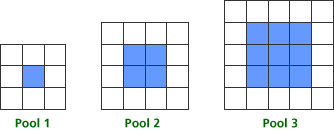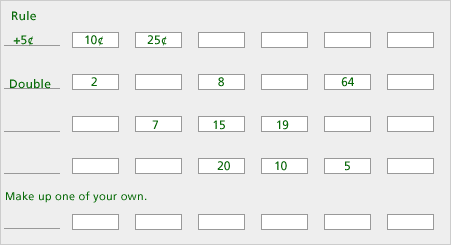# Classroom Case Studies, Grades 3-5 Part B: An Example for Developing Algebraic Thinking (20 minutes)

## Session 10: 3-5, Part B

The National Council of Teachers of Mathematics’ Principles and Standards for School Mathematics (2000) identifies algebra as a strand for grades Pre-K-12. The Standardsidentify the following concepts that all students should cover and comprehend:
Note 4

 • Understand patterns, relationships, and functions • Represent and analyze mathematical situations and structures using algebraic symbols • Use mathematical models to represent and understand quantitative relationships • Analyze change in various contexts

For the classroom in Grade 3-5, understanding patterns includes the following expectations:

 • Describe, extend, and make generalizations about geometric and numeric patterns • Represent and analyze patterns and functions, using words, tables, and graphs • Represent the idea of a variable as an unknown quantity using a letter or a symbol

In this part, we’ll look at problems that foster algebraic thinking as it relates to these standards, and we’ll explore ways of asking questions that elicit algebraic thinking. The situations we will be exploring are representative of the kinds of problems you would find in some existing texts; in fact, you may recognize some of them! The goal is for you to examine these problems with the critical eye of someone who has taken this course and is beginning to view algebraic thinking with a different perspective.

Consider the situation below, appropriate for exploration in a Grade 3-5 classroom:

Tat Ming is designing square swimming pools. Each pool has a square center that is the area of the water. Tat Ming uses blue tiles to represent the water. Around each pool there is a border of white tiles. Here are pictures of the three smallest square pools that he can design, with blue tiles for the interior and white tiles for the border. Note 4### Problems

Problem B1

Problem B2

How do your questions reflect the algebra content in the situation?

Now focus on the questions you want the students in your classroom to consider. You may want to begin by having them draw a table that shows the pool “number” and the relative number of blue and white tiles. Students may also want to begin by looking at the fraction of blue or white tiles out of the whole number of tiles.

Problem B3

What patterns, conjectures, and questions will your students find as they work with this situation?

Problem B4

What questions could you as the teacher pose to elicit and extend student thinking at your grade level?

Problem B5

Recall the framework you explored in Session 2 in looking at patterns: finding, describing, explaining, and using patterns to predict. Which of these skills will your students use in approaching this problem?

Problem B6

Read the article “Experiences with Patterning” from Teaching Children Mathematics.What ideas mentioned seem appropriate for your classroom? Note 5

Here is a set of problems on patterns:

 37, 40, 43, _____, _____, _____ 27, 25, _____, 21, _____, _____ _____, 11, 15, _____, 23, _____ _____, _____, 36, 33, _____, 27Problem B7

What questions could you ask to develop students’ skills in describing these patterns?

Problem B8

What questions could you ask to develop students’ skills in predicting?

### Notes

Note 4

Look at NCTM’s recommendations for content in the algebra strand in the Standards,then look at the problem for designing square swimming pools. After reading the problem, work on Problems B1-B5.

Note 5

Read the commentary on the swimming pool problem in “Experiences with Patterning,” by Joan Ferrini-Mundy, Glenda Lappan, and Elizabeth Phillips, in Teaching Children Mathematics (February 1997), p. 282-288.

Now look at the problems on patterns from Everyday Mathematics, Student Journal.Think of questions that extend students’ thinking beyond simply finding the next number:

 • Could you predict the 20th number in the list? • Could 11 ever be a number in the list? • If you knew the 99th number, could you determine the 101st number?

### Solutions

Problem B1

Answers will vary. One question might ask how to use the pictures to help find the relationship between the pool number and the numbers of white and blue tiles

Problem B2

Answers will vary. For the question above, you might discuss building each pool. For example, the blue part of Pool 1 is a 1-by-1 square and takes one blue tile, the blue part of Pool 2 is a 2-by-2 square and takes four blue tiles, etc.

Problem B3

Answers will vary. At this level, many students will see that the blue tiles are always in the shape of a square, and that there are always an even number of white tiles. Some may recognize that the number of white tiles is always a multiple of four.

Problem B4

Answers will vary. At this level, teachers should encourage students to describe the shape of the blue tiles and write a rule to represent the relationship between the pool number and the number of blue tiles. Then ask them to think about putting white tiles around that blue pool. How many white tiles do you need for the top? How many for the bottom? Now think about the putting tiles around the sides. How many white tiles does this require in all?

Problem B5

Answers will vary. All of the ideas described in the grades 3-4 section are appropriate for this level student. Many students at this level will be able to answer questions posed in the grades 5-6 section.

Problem B6

Answers will vary. All of the ideas described in the grades 3-4 section are appropriate for this level student. Many students at this level will be able to answer questions posed in the grades 5-6 section.

Problem B7

Answers will vary. Ask students to look at two consecutive entries and think of ways to get from one to the other. Then have students continue that pattern to see if other entries are correct.

Problem B8

Answers will vary. Often students see a rule to get from one entry to the next. Teachers should help students think of ways to connect each entry to its place in the list.